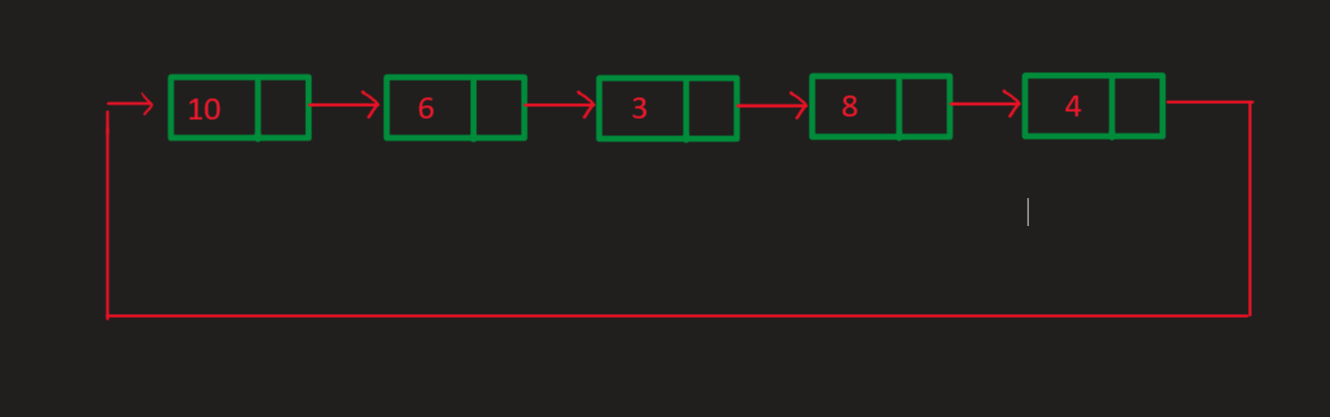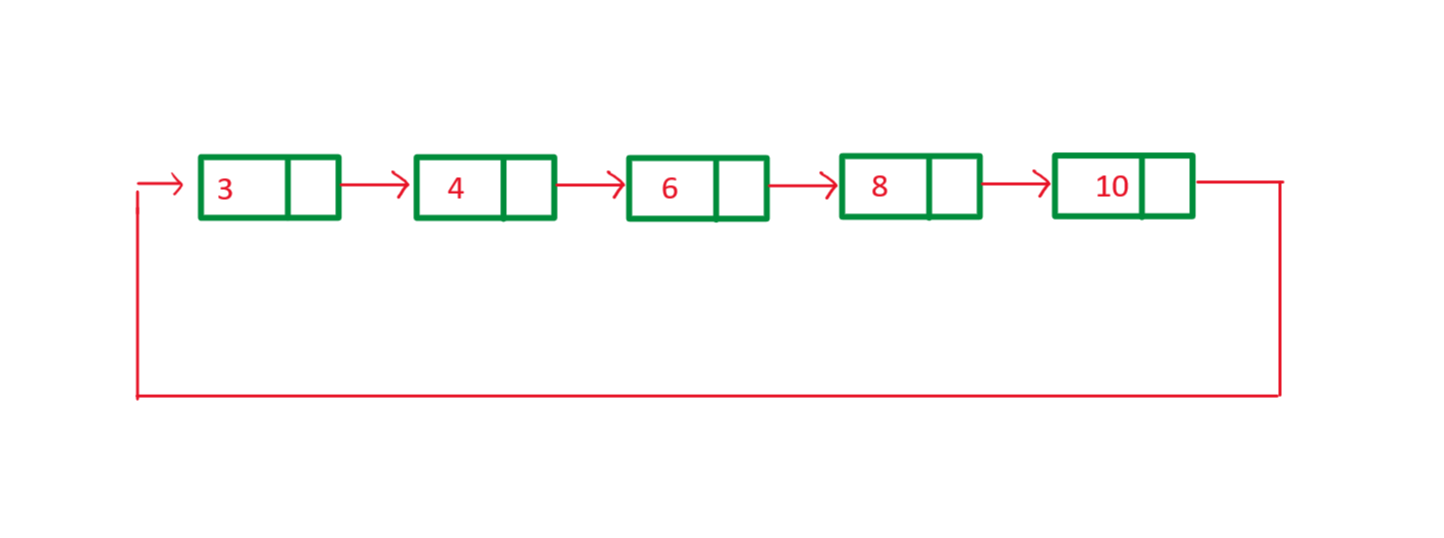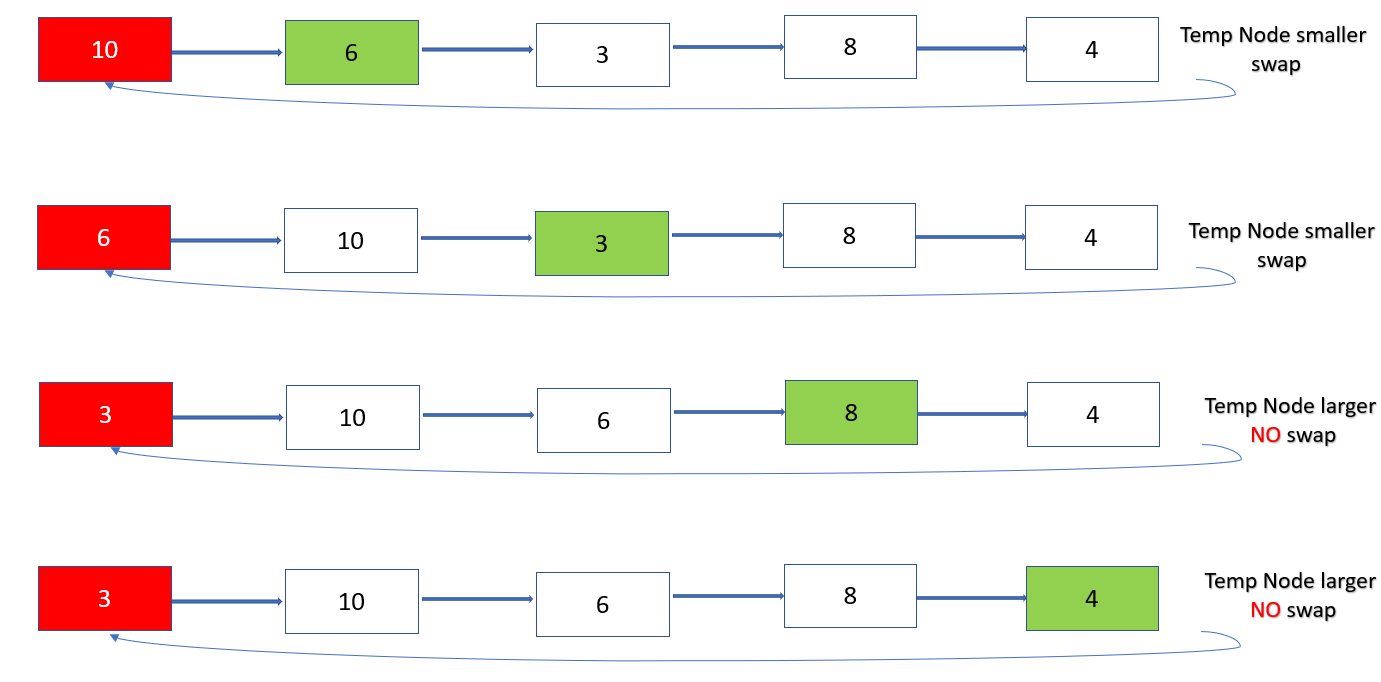Related Articles
Java Program to Sort the Elements of the Circular Linked List
• Difficulty Level : Hard
• Last Updated : 11 Dec, 2020

In a circular linked list, every node points to its next node in the sequence but the last node points to the first node in the list. Here, Create a circular linked list and sort the circular linked list in ascending order.Approach:

1. Take two pointers: Current pointing to head of the node and Temp pointing to next node of Current.
2. Now for each iteration compare value of Current pointer to the value of Temp pointer.
Here two cases arise

Case 1: If the value of a current pointer is greater than that of Temp pointer
Swap values of a current pointer and temp pointer.
Move the temp pointer to next node
Case 2: If the value of a current pointer is less than or equal to that of Temp pointer
Move the temp pointer to next node

3. Now keep doing this until temp.next !=head of the list.
4. After completing step 3 move the Current to next node and repeat the steps 1,2,3 .
5. Each iteration results in fixing of the shortest element of the list to it’s correct position.
6. Repeat the above steps until Current. Next != head of list .

Let’s see how this work for the first node of the given circular linked listBelow is the implementation of the above approach:

## Java

 `// Java Program to Sort the Elements``// of the Circular Linked List`` ` `import` `java.io.*;`` ` `public` `class` `GFG {``    ``// Stores Information about Node of List``    ``public` `class` `Node {``        ``int` `data;``        ``Node next;``        ``public` `Node(``int` `data) { ``this``.data = data; }``    ``}`` ` `    ``// Declaring Head of the Node``    ``public` `Node head_of_node = ``null``;`` ` `    ``// A last pointer to help append values to our list``    ``public` `Node last = ``null``;`` ` `    ``// Add method adds values to the end of the list``    ``public` `void` `add(``int` `data)``    ``{``        ``Node newNode = ``new` `Node(data);``        ``if` `(head_of_node == ``null``) {``            ``head_of_node = newNode;``            ``last = newNode;``            ``newNode.next = head_of_node;``        ``}``        ``else` `{``            ``last.next = newNode;``            ``last = newNode;``            ``last.next = head_of_node;``        ``}``    ``}``    ``// Sort_List method sorts the circular``    ``// linked list Using the algorithm``    ``public` `void` `Sort_List()``    ``{`` ` `        ``// current pointer pointing to the head of the list``        ``Node current = head_of_node;`` ` `        ``// a temp pointer``        ``Node temp = ``null``;`` ` `        ``// variable value helps in swap of the values``        ``int` `value;`` ` `        ``// this is the Algorithm discussed above``        ``if` `(head_of_node == ``null``) {``            ``System.out.println(``"Your list is empty"``);``        ``}``        ``else` `{``            ``while` `(current.next != head_of_node) {``                ``temp = current.next;``                ``while` `(temp != head_of_node) {``                    ``if` `(current.data > temp.data) {``                        ``value = current.data;``                        ``current.data = temp.data;``                        ``temp.data = value;``                    ``}``                    ``temp = temp.next;``                ``}``                ``current = current.next;``            ``}``        ``}``    ``}``    ``// Print_list method iterates through the list and``    ``// prints the values stored in the list``    ``public` `void` `Print_List()``    ``{``        ``Node current = head_of_node;``        ``if` `(head_of_node == ``null``) {``            ``System.out.println(``"Your list is empty"``);``        ``}``        ``else` `{``            ``do` `{``                ``System.out.print(``" "` `+ current.data);``                ``current = current.next;``            ``} ``while` `(current != head_of_node);``            ``System.out.println();``        ``}``    ``}`` ` `    ``// Driver code``    ``public` `static` `void` `main(String[] args)``    ``{`` ` `        ``GFG circular_list = ``new` `GFG();``        ``circular_list.add(``10``);``        ``circular_list.add(``6``);``        ``circular_list.add(``3``);``        ``circular_list.add(``8``);``        ``circular_list.add(``4``);`` ` `        ``System.out.print(``"Original List -->     "``);``        ``circular_list.Print_List();``        ``circular_list.Sort_List();``        ``System.out.print(``"List after Sorting--> "``);``        ``circular_list.Print_List();``    ``}``}`
Output
```Original List -->      10 6 3 8 4
List after Sorting-->  3 4 6 8 10```

Time Complexity: O(N2)

Attention reader! Don’t stop learning now. Get hold of all the important Java Foundation and Collections concepts with the Fundamentals of Java and Java Collections Course at a student-friendly price and become industry ready. To complete your preparation from learning a language to DS Algo and many more,  please refer Complete Interview Preparation Course.

My Personal Notes arrow_drop_up